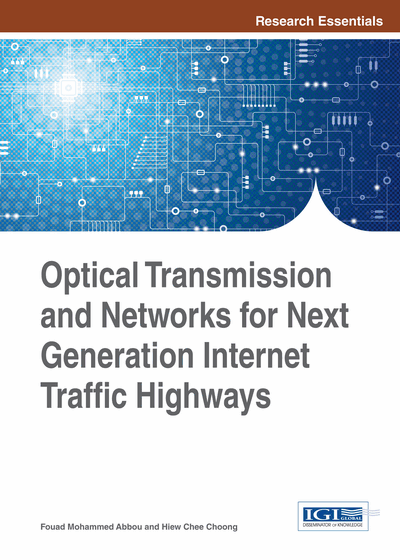# Optical Transport Network: A Physical Layer Perspective Part 2

DOI: 10.4018/978-1-4666-6575-0.ch002
Available
\$37.50
No Current Special Offers

## Abstract

The purpose of this chapter is to discuss two major categories of nonlinear effects related either to nonlinear refractive index or to nonlinear stimulated scattering effects. The effects related to nonlinear refractive index occur due to the dependence of the refractive index on the optical signal intensity. On the other hand, stimulated scattering effects are caused by interaction between light and material. As many wavelength signal channels travel through the optical fiber, they encounter many of those nonlinear effects impairments that affect the signal power level and hence degrade sharply the quality of the signal resulting in interference among signals carried by different wavelength channels. Therefore, before delving into the optical transmission performance issues, a physical picture of how optical signals behave in the presence of the most important nonlinear effect impairments is drawn.
Chapter Preview
Top

## Self Phase Modulation

Self phase modulation (SPM) is the nonlinear effect caused by the change of the nonlinear refractive index due to the intensity of the incident signal on itself. This means that the power of the signal acts to alter the refractive index of the fiber causing a phase modulation on the signal. This would not cause any problems if the intensity of the signal is constant as it would then only induce a constant phase shift over the entire signal. However, for an intensity modulated system, the signal intensity is not constant, but changes according to the modulation guided by bit sequence, thus generating phase shifts that change along the signal. These phase shifts occur almost instantaneously and are dependent on the current signal intensity because the nonlinear phase shift is an ultrafast process. Thus, any fluctuations in intensity create an almost instant fluctuation in phase.

The induced phase shift, ϕSPM(z,T) is distance and time dependent and can be approximated for a single fiber configuration by (Uetsuka, 2004)

(2.2) where E(0,T) is the signal field envelope at distance z = 0, α is the fiber loss (attenuation) parameter, P0 is the pulse peak power and γ is the nonlinearity coefficient given by(2.3) where n2 is the nonlinear refractive index, ω0 is the signal center frequency and Aeff is the fiber effective area. From (Equation (2.2)), we can see that the SPM induced phase shift increases with power and distance, but the distance factor is limited by the exponential term associated with the fiber attenuation. In fact, for a distance L, the effective distance is defined as

Leff =(2.4)

## Complete Chapter List

Search this Book:
Reset